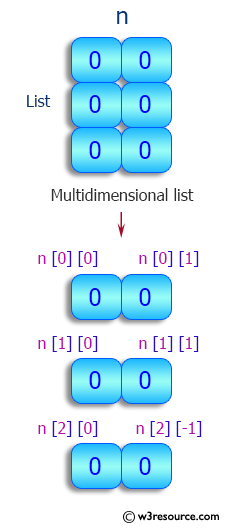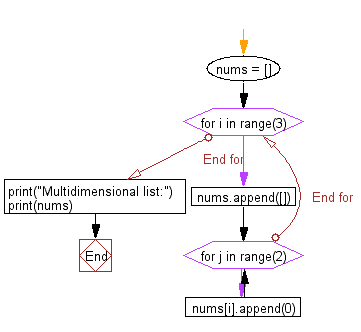﻿ Python: Create a multidimensional list with zeros - w3resource# Python: Create a multidimensional list with zeros

## Python List: Exercise - 85 with Solution

Write a Python program to create a multidimensional list (lists of lists) with zeros.

Sample Solution:

Python Code:

``````nums = []

for i in range(3):

nums.append([])

for j in range(2):

nums[i].append(0)
print("Multidimensional list:")
print(nums)
```
```

Sample Output:

```Multidimensional list:
[[0, 0], [0, 0], [0, 0]]
```

Pictorial Presentation:Flowchart:## Visualize Python code execution:

The following tool visualize what the computer is doing step-by-step as it executes the said program:

Python Code Editor:

Have another way to solve this solution? Contribute your code (and comments) through Disqus.

What is the difficulty level of this exercise?

Test your Python skills with w3resource's quiz

﻿

## Python: Tips of the Day

Floor Division:

When we speak of division we normally mean (/) float division operator, this will give a precise result in float format with decimals.

For a rounded integer result there is (//) floor division operator in Python. Floor division will only give integer results that are round numbers.

```print(1000 // 300)
print(1000 / 300)```

Output:

```3
3.3333333333333335```